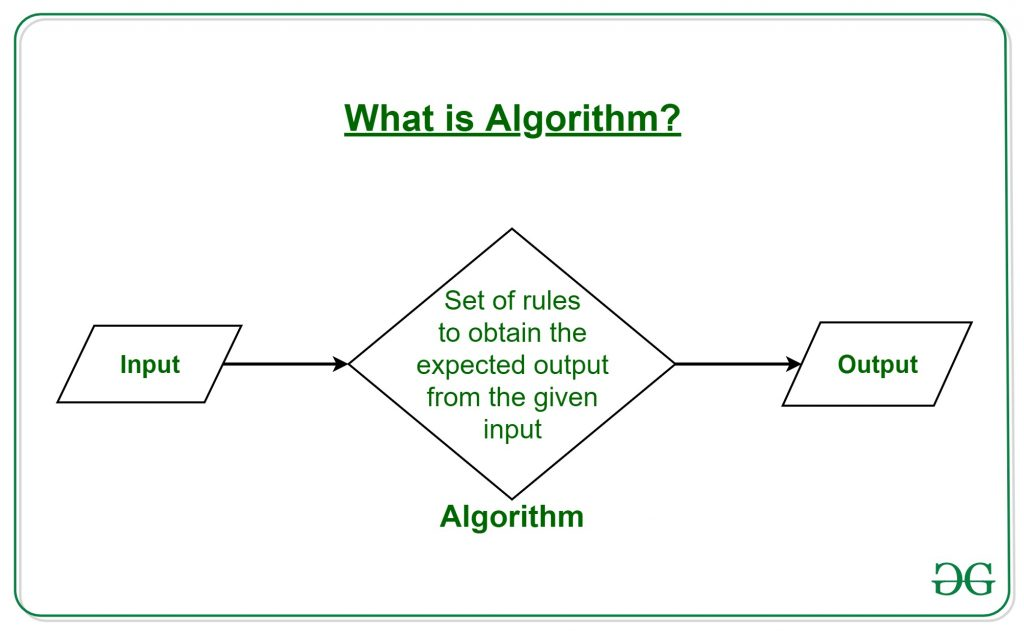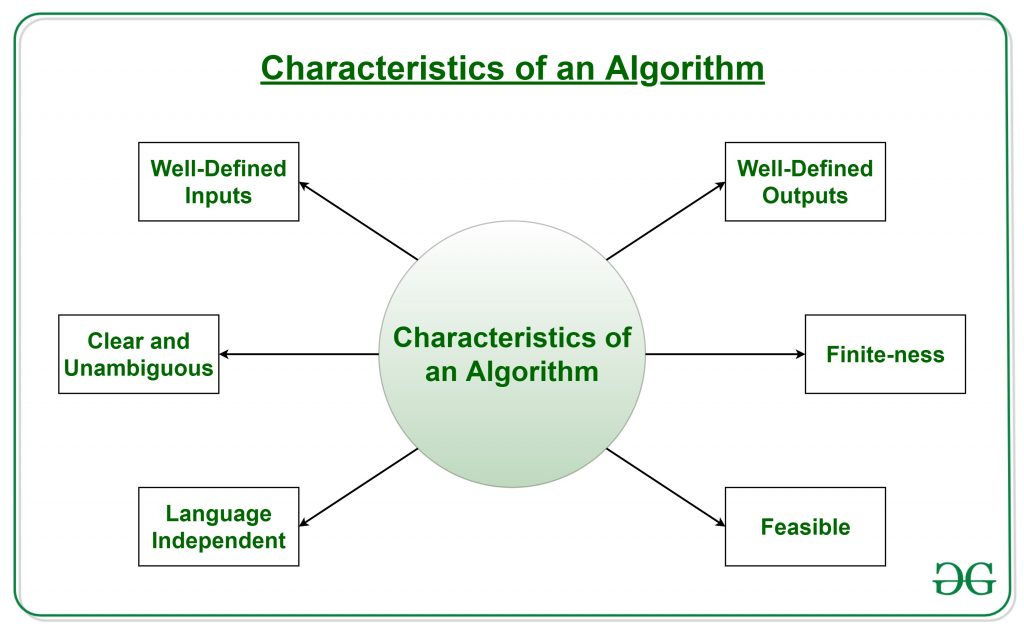# Introduction to Algorithms

### What is Algorithm? Algorithm Basics

The word Algorithm means “a process or set of rules to be followed in calculations or other problem-solving operations”. Therefore Algorithm refers to a set of rules/instructions that step-by-step define how a work is to be executed upon in order to get the expected results.It can be understood by taking an example of cooking a new recipe. To cook a new recipe, one reads the instructions and steps and execute them one by one, in the given sequence. The result thus obtained is the new dish cooked perfectly. Similarly, algorithms help to do a task in programming to get the expected output.
The Algorithm designed are language-independent, i.e. they are just plain instructions that can be implemented in any language, and yet the output will be the same, as expected.

### What are the Characteristics of an Algorithm?As one would not follow any written instructions to cook the recipe, but only the standard one. Similarly, not all written instructions for programming is an algorithm. In order for some instructions to be an algorithm, it must have the following characteristics:

• Clear and Unambiguous: Algorithm should be clear and unambiguous. Each of its steps should be clear in all aspects and must lead to only one meaning.
• Well-Defined Inputs: If an algorithm says to take inputs, it should be well-defined inputs.
• Well-Defined Outputs: The algorithm must clearly define what output will be yielded and it should be well-defined as well.
• Finite-ness: The algorithm must be finite, i.e. it should not end up in an infinite loops or similar.
• Feasible: The algorithm must be simple, generic and practical, such that it can be executed upon will the available resources. It must not contain some future technology, or anything.
• Language Independent: The Algorithm designed must be language-independent, i.e. it must be just plain instructions that can be implemented in any language, and yet the output will be same, as expected.

• It is easy to understand.
• Algorithm is a step-wise representation of a solution to a given problem.
• In Algorithm the problem is broken down into smaller pieces or steps hence, it is easier for the programmer to convert it into an actual program.

• Writing an algorithm takes a long time so it is time-consuming.
• Branching and Looping statements are difficult to show in Algorithms.

### How to Design an Algorithm?

In order to write an algorithm, following things are needed as a pre-requisite:

1. The problem that is to be solved by this algorithm.
2. The constraints of the problem that must be considered while solving the problem.
3. The input to be taken to solve the problem.
4. The output to be expected when the problem the is solved.
5. The solution to this problem, in the given constraints.

Then the algorithm is written with the help of above parameters such that it solves the problem.
Example: Consider the example to add three numbers and print the sum.

• Step 1: Fulfilling the pre-requisites
As discussed above, in order to write an algorithm, its pre-requisites must be fulfilled.
1. The problem that is to be solved by this algorithm: Add 3 numbers and print their sum.
2. The constraints of the problem that must be considered while solving the problem: The numbers must contain only digits and no other characters.
3. The input to be taken to solve the problem: The three numbers to be added.
4. The output to be expected when the problem the is solved: The sum of the three numbers taken as the input.
5. The solution to this problem, in the given constraints: The solution consists of adding the 3 numbers. It can be done with the help of ‘+’ operator, or bit-wise, or any other method.
• Step 2: Designing the algorithm
Now let’s design the algorithm with the help of above pre-requisites:
Algorithm to add 3 numbers and print their sum:
1. START
2. Declare 3 integer variables num1, num2 and num3.
3. Take the three numbers, to be added, as inputs in variables num1, num2, and num3 respectively.
4. Declare an integer variable sum to store the resultant sum of the 3 numbers.
5. Add the 3 numbers and store the result in the variable sum.
6. Print the value of variable sum
7. END
• Step 3: Testing the algorithm by implementing it.
Inorder to test the algorithm, let’s implement it in C language.

Program:

## C++

 `// C++ program to add three numbers` `// with the help of above designed ` `// algorithm` `#include ` `using` `namespace` `std;` ` `  `int` `main()` `{` `    `  `    ``// Variables to take the input of ` `    ``// the 3 numbers` `    ``int` `num1, num2, num3;` ` `  `    ``// Variable to store the resultant sum` `    ``int` `sum;` ` `  `    ``// Take the 3 numbers as input` `    ``cout << ``"Enter the 1st number: "``;` `    ``cin >> num1;` `    ``cout << ``" "` `<< num1 << endl;` ` `  `    ``cout << ``"Enter the 2nd number: "``;` `    ``cin >> num2;` `    ``cout << ``" "` `<< num2 << endl;` ` `  `    ``cout << ``"Enter the 3rd number: "``;` `    ``cin >> num3;` `    ``cout << ``" "` `<< num3;` ` `  `    ``// Calculate the sum using + operator` `    ``// and store it in variable sum` `    ``sum = num1 + num2 + num3;` ` `  `    ``// Print the sum` `    ``cout << ``"\nSum of the 3 numbers is: "` `         ``<< sum;` ` `  `    ``return` `0;` `}`   `// This code is contributed by shivanisinghss2110`

## C

 `// C program to add three numbers` `// with the help of above designed algorithm`   `#include `   `int` `main()` `{`   `    ``// Variables to take the input of the 3 numbers` `    ``int` `num1, num2, num3;`   `    ``// Variable to store the resultant sum` `    ``int` `sum;`   `    ``// Take the 3 numbers as input` `    ``printf``(``"Enter the 1st number: "``);` `    ``scanf``(``"%d"``, &num1);` `    ``printf``(``"%d\n"``, num1);`   `    ``printf``(``"Enter the 2nd number: "``);` `    ``scanf``(``"%d"``, &num2);` `    ``printf``(``"%d\n"``, num2);`   `    ``printf``(``"Enter the 3rd number: "``);` `    ``scanf``(``"%d"``, &num3);` `    ``printf``(``"%d\n"``, num3);`   `    ``// Calculate the sum using + operator` `    ``// and store it in variable sum` `    ``sum = num1 + num2 + num3;`   `    ``// Print the sum` `    ``printf``(``"\nSum of the 3 numbers is: %d"``, sum);`   `    ``return` `0;` `}`

Output

```Enter the 1st number: 0
Enter the 2nd number: 0
Enter the 3rd number: -1577141152

Sum of the 3 numbers is: -1577141152```
• + operator
• Bit-wise operators
• . . etc
1. Priori Analysis: “Priori” means “before”. Hence Priori analysis means checking the algorithm before its implementation. In this, the algorithm is checked when it is written in the form of theoretical steps. This Efficiency of an algorithm is measured by assuming that all other factors, for example, processor speed, are constant and have no effect on the implementation. This is done usually by the algorithm designer. It is in this method, that the Algorithm Complexity is determined.
2. Posterior Analysis: “Posterior” means “after”. Hence Posterior analysis means checking the algorithm after its implementation. In this, the algorithm is checked by implementing it in any programming language and executing it. This analysis helps to get the actual and real analysis report about correctness, space required, time consumed etc.
• Time Factor: Time is measured by counting the number of key operations such as comparisons in the sorting algorithm.
• Space Factor: Space is measured by counting the maximum memory space required by the algorithm.
1. Space Complexity: Space complexity of an algorithm refers to the amount of memory that this algorithm requires to execute and get the result. This can be for inputs, temporary operations, or outputs.
How to calculate Space Complexity?
The space complexity of an algorithm is calculated by determining following 2 components:
• Fixed Part: This refers to the space that is definitely required by the algorithm. For example, input variables, output variables, program size, etc.
• Variable Part: This refers to the space that can be different based on the implementation of the algorithm. For example, temporary variables, dynamic memory allocation, recursion stack space, etc.
2. Time Complexity: Time complexity of an algorithm refers to the amount of time that this algorithm requires to execute and get the result. This can be for normal operations, conditional if-else statements, loop statements, etc.
How to calculate Time Complexity?
The time complexity of an algorithm is also calculated by determining following 2 components:
• Constant time part: Any instruction that is executed just once comes in this part. For example, input, output, if-else, switch, etc.
• Variable Time Part: Any instruction that is executed more than once, say n times, comes in this part. For example, loops, recursion, etc.

Attention reader! Don’t stop learning now. Get hold of all the important DSA concepts with the DSA Self Paced Course at a student-friendly price and become industry ready.

My Personal Notes arrow_drop_upContent Manager at LambdaTest

If you like GeeksforGeeks and would like to contribute, you can also write an article using contribute.geeksforgeeks.org or mail your article to contribute@geeksforgeeks.org. See your article appearing on the GeeksforGeeks main page and help other Geeks.

Please Improve this article if you find anything incorrect by clicking on the "Improve Article" button below.